# ISEE Middle Level Math : How to find the area of a rectangle

## Example Questions

### Example Question #41 : Rectangles

Use the following image to answer the question: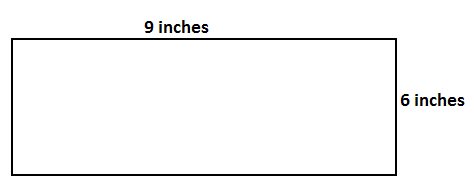Find the area of the rectangle.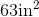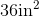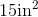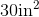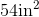Explanation:

To find the area of a rectangle, we will use the following formula: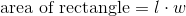where l is the length and w is the width of the rectangle.

Now, let's look at the rectangle.We can see the length is 9 inches, and the width is 6 inches.

Knowing this, we can substitute into the formula.  We get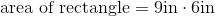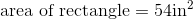### Example Question #41 : Rectangles

Find the area of a rectangle with a width of 4in and a length that is three times the width.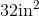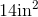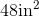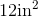Explanation:

To find the area of a rectangle, we will use the following formula:where l is the length and w is the width of the rectangle.

Now, we know the width of the rectangle is 4in.  We also know the length of the rectangle is three times the width.  Therefore, the length is 12in.

Knowing this, we can substitute into the formula.  We get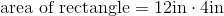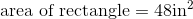### Example Question #42 : Rectangles

Find the area of a rectangle with a width of 9cm and a length that is two times the width.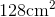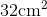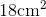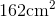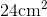Explanation:

To find the area of a rectangle, we will use the following formula:where l is the length and w is the width of the rectangle.

Now, we know the width of the rectangle is 9cm.  We also know the length of the rectangle is two times the width.  Therefore, the length is 18cm.

Knowing this, we will substitute into the formula.  We get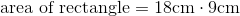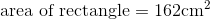### Example Question #41 : Rectangles

Find the area of a rectangle with a width of 4in and a length that is three times the width.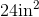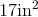Explanation:

To find the area of a rectangle, we will use the following formula:where l is the length and w is the width of the rectangle.

Now, we know the width of the rectangle is 4in.  We also know the length is three times the width.  Therefore, the length is 12in.

Knowing this, we can substitute into the formula.  We get### Example Question #43 : Rectangles

Find the area of a rectangle with a width of 3cm and a length that is three times the width.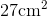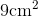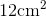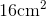Explanation:

To find the area of a rectangle, we will use the following formula:where is the length and w is the width of the rectangle.

So, we know the width of the rectangle is 3cm.  We also know the length is three times the width.  Therefore, the length is 9cm.

Knowing this, we can substitute into the formula.  We get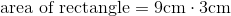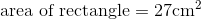### Example Question #46 : Rectangles

Find the area of a rectangle with a length of 12cm and a width that is a quarter of the length.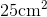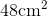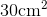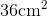Explanation:

To find the area of a rectangle, we will use the following formula:where l is the length and w is the width of the rectangle.

Now, we know the length of the rectangle is 12cm.  We also know the width of the rectangle is a quarter of the length.  To find the width, we will divide 12 by 4.  Therefore, the width the 3cm.

Knowing this, we will substitute into the formula.  We get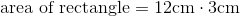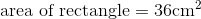### Example Question #44 : Rectangles

Find the area of a rectangle with a width of 8in and a length that is two times the width.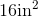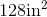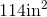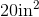Explanation:

To find the area of a rectangle, we will use the following formula:where l is the length and w is the width of the rectangle.

Now, we know the width of the rectangle is 8in.  We also know the length is two times the width.  Therefore, the length is 16in.

Knowing this, we can substitute into the formula.  We get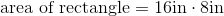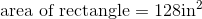### Example Question #43 : Rectangles

Find the area of a rectangle with a length of 24in and a width that is a third of the length.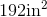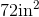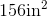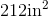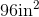Explanation:

To find the area of a rectangle, we will use the following formula: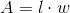where l is the length and w is the width of the rectangle.

Now, we know the length of the rectangle is 24in.  We also know the width is a third of the length.  Therefore, the width is 8in.

Knowing this, we can substitute into the formula.  We get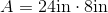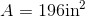### Example Question #46 : Rectangles

Find the area of a rectangle with a width of 6in and a length that is four times the width.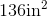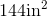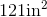Explanation:

To find the area of a rectangle, we will use the following formula:where l is the length and w is the width of the rectangle.

Now, we know the width of the rectangle is 6in.  We also know the length is four times the width.  Therefore, the length is 24in.

Knowing this, we can substitute into the formula.  We get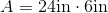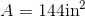### Example Question #47 : Rectangles

Find the area of a rectangle with a length of 21in and a width that is a third of the length.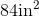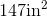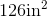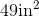Explanation:

To find the area of a rectangle, we will use the following formula:where l is the length and w is the width of the rectangle.

We know the length of the rectangle is 21in.  We also know the width of the rectangle is a third of the length.  Therefore, the width is 7in.

Knowing this, we can substitute into the formula.  We get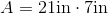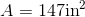### All ISEE Middle Level Math Resources